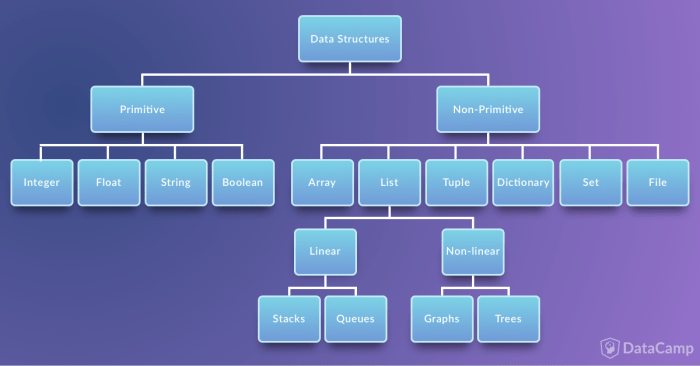# Python学习教程_Python学习路线：Python数据结构

Python学习教程_Python学习路线：Python数据结构• Integers
• Float
• Strings
• Boolean

Integers

Float

“Float”代表“浮点数”。 您可以将它用于有理数，通常以十进制数字结尾，例如1.11或3.14。

String

`x = 'Cake'y = 'Cookie'x + ' & ' + y #结果:'Cake & Cookie'`

`# Repeatx * 2 #结果：'CakeCake'`

`z1 = x[2:] print(z1)z2 = y + y print(z2)# 结果keCo`

`x = '4'y = '2'x + y'42'`

Python有许多内置方法或辅助函数来操作字符串。 替换子字符串，大写段落中的某些单词，在另一个字符串中查找字符串的位置是一些常见的字符串操作。 例如：

• 大写首字母
`str.capitalize('cookie')'Cookie'`
• 以字符为单位检索字符串的长度。 请注意，空格也计入最终结果：
`str1 = "Cake 4 U"str2 = "404"len(str1)8`
• 检查字符串是否数字
`str1 = "Cake 4 U"str2 = "404"str1.isdigit()Falsestr2.isdigit()True`
• 替换
`str1 = "Cake 4 U"str2 = "404"str1.replace('4 U', str2)'Cake 404'`
• 查找子字符串
`str1 = 'cookie'str2 = 'cook'str1.find(str2)0str1 = 'I got you a cookie'str2 = 'cook'str1.find(str2)12`

Boolean

`x = 4y = 2x == yFalsex > yTruex = 4y = 2z = (x==y)if z: print("Cookie")else: print("No Cookie")No Cookie`

`i = 4.0type(i)float`

`# floatx = 4.0 # integery = 2 z = x/ytype(z)float`

`x = 2y = "The Godfather: Part "fav_movie = y + xTypeErrorTraceback (most recent call last)<ipython-input-51-b8fe90df9e0e> in <module>() 1 x = 2 2 y = "The Godfather: Part "----> 3 fav_movie = y + xTypeError: Can't convert 'int' object to str implicitly`

`x = 2y = "The Godfather: Part "fav_movie = (y) + str(x)print(fav_movie)The Godfather: Part 2`

python内置了一些类型转换函数：int(), float(), 和 str()

• Arrays
• Lists
• Files

Arrays

`import array as arra = arr.array("I",[3,6,9])type(a)array.array`

Lists

Python中的列表用于存储同类项的集合。 这些是可变的，这意味着您可以在不改变其身份的情况下更改其内容。 您可以通过方括号[和]来识别列表，其中包含以逗号分隔的元素。 列表内置于Python中：您无需单独调用它们。

`x = []type(x)listx1 = [1,2,3]type(x1)listx2 = list([1,'apple',3])type(x2)listprint(x2)applex2 = 'orange'print(x2)[1, 'orange', 3]`

Python提供了许多操作和处理列表的方法。 将新项添加到列表，从列表中删除一些项，排序或反转列表是常见的列表操作。 例如：

• 使用append（）将11添加到list_num列表中。 默认情况下，此数字将添加到列表的末尾。
`list_num = [1,2,45,6,7,2,90,23,435]list_char = ['c','o','o','k','i','e']list_num.append(11) print(list_num)[1, 2, 45, 6, 7, 2, 90, 23, 435, 11]`
• 插入数据
`list_num.insert(0, 11)print(list_num)[11, 1, 2, 45, 6, 7, 2, 90, 23, 435, 11]`
• 删除元素
`list_char.remove('o') print(list_char)['c', 'o', 'k', 'i', 'e']`
• 根据索引删除元素
`list_char.pop(-2) # Removes the item at the specified positionprint(list_char)['c', 'o', 'k', 'e']list_num.sort()print(list_num)[1, 2, 2, 6, 7, 11, 11, 23, 45, 90, 435]list.reverse(list_num)print(list_num)[435, 90, 45, 23, 11, 11, 7, 6, 2, 2, 1]`

Array vs List

`array_char = array.array("u",["c","a","t","s"])array_char.tostring()print(array_char)array('u', 'cats')`

`import numpy as nparr_a = np.array([3, 6, 9])arr_b = arr_a/3 # Performing vectorized (element-wise) operations print(arr_b)[ 1. 2. 3.]arr_ones = np.ones(4)print(arr_ones)[ 1. 1. 1. 1.]multi_arr_ones = np.ones((3,4)) print(multi_arr_ones)[[ 1. 1. 1. 1.] [ 1. 1. 1. 1.] [ 1. 1. 1. 1.]]`

Stack

`# Bottom -> 1 -> 2 -> 3 -> 4 -> 5 (Top)stack = [1,2,3,4,5] stack.append(6) # Bottom -> 1 -> 2 -> 3 -> 4 -> 5 -> 6 (Top)print(stack)[1, 2, 3, 4, 5, 6]stack.pop() # Bottom -> 1 -> 2 -> 3 -> 4 -> 5 (Top)stack.pop() # Bottom -> 1 -> 2 -> 3 -> 4 (Top)print(stack)[1, 2, 3, 4]`

Queue

Graphs

`graph = { "a" : ["c", "d"], "b" : ["d", "e"], "c" : ["a", "e"], "d" : ["a", "b"], "e" : ["b", "c"] }def define_edges(graph): edges = [] for vertices in graph: for neighbour in graph[vertices]: edges.append((vertices, neighbour)) return edgesprint(define_edges(graph))[('a', 'c'), ('a', 'd'), ('b', 'd'), ('b', 'e'), ('c', 'a'), ('c', 'e'), ('e', 'b'), ('e', 'c'), ('d', 'a'), ('d', 'b')]`

Tree`class Tree: def __init__(self, info, left=None, right=None): self.info = info self.left = left self.right = right def __str__(self): return (str(self.info) + ', Left child: ' + str(self.left) + ', Right child: ' + str(self.right))tree = Tree(1, Tree(2, 2.1, 2.2), Tree(3, 3.1))print(tree)1, Left child: 2, Left child: 2.1, Right child: 2.2, Right child: 3, Left child: 3.1, Right child: None`

Tuples

`x_tuple = 1,2,3,4,5y_tuple = ('c','a','k','e')x_tuple1y_tuplex_tuple = 0 # Cannot change values inside a tuple---------------------------------------------------------------------------TypeErrorTraceback (most recent call last)<ipython-input-74-b5d6da8c1297> in <module>() 1 y_tuple----> 2 x_tuple = 0 # Cannot change values inside a tupleTypeError: 'tuple' object does not support item assignment`

Dictionary

`x_dict = {'Edward':1, 'Jorge':2, 'Prem':3, 'Elisa':4}del x_dict['Elisa']x_dict{'Edward': 1, 'Jorge': 2, 'Prem': 3}x_dict['Edward'] # Prints the value stored with the key 'Kevin'.1`

`len(x_dict)3x_dict.keys()dict_keys(['Prem', 'Edward', 'Jorge'])x_dict.values()dict_values([3, 1, 2])`

Sets

`x_set = set('CAKE&COKE')y_set = set('COOKIE')print(x_set){'A', '&', 'O', 'E', 'C', 'K'}print(y_set) # Single unique 'o'{'I', 'O', 'E', 'C', 'K'}print(x - y) # All the elements in x_set but not in y_set---------------------------------------------------------------------------NameError Traceback (most recent call last)<ipython-input-3-31abf5d98454> in <module>()----> 1 print(x - y) # All the elements in x_set but not in y_setNameError: name 'x' is not definedprint(x_set|y_set) # Unique elements in x_set or y_set or both{'C', '&', 'E', 'A', 'O', 'K', 'I'}print(x_set & y_set) # Elements in both x_set and y_set{'O', 'E', 'K', 'C'}`

Files

• open（）打开系统中的文件，文件名是要打开的文件的名称;
`# File modes (2nd argument): 'r'(read), 'w'(write), 'a'(appending), 'r+'(both reading and writing)f = open('file_name', 'w')# Reads entire filef.read() # Reads one line at a timef.readline() # Writes the string to the file, returning the number of char writtenf.write('Add this line.') f.close()`